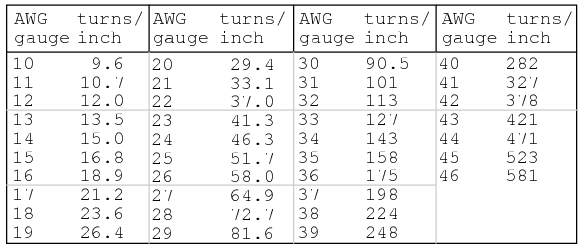# 16.6: Inductor Sizing Equation

$$\newcommand{\vecs}{\overset { \rightharpoonup} {\mathbf{#1}} }$$ $$\newcommand{\vecd}{\overset{-\!-\!\rightharpoonup}{\vphantom{a}\smash {#1}}}$$$$\newcommand{\id}{\mathrm{id}}$$ $$\newcommand{\Span}{\mathrm{span}}$$ $$\newcommand{\kernel}{\mathrm{null}\,}$$ $$\newcommand{\range}{\mathrm{range}\,}$$ $$\newcommand{\RealPart}{\mathrm{Re}}$$ $$\newcommand{\ImaginaryPart}{\mathrm{Im}}$$ $$\newcommand{\Argument}{\mathrm{Arg}}$$ $$\newcommand{\norm}{\| #1 \|}$$ $$\newcommand{\inner}{\langle #1, #2 \rangle}$$ $$\newcommand{\Span}{\mathrm{span}}$$ $$\newcommand{\id}{\mathrm{id}}$$ $$\newcommand{\Span}{\mathrm{span}}$$ $$\newcommand{\kernel}{\mathrm{null}\,}$$ $$\newcommand{\range}{\mathrm{range}\,}$$ $$\newcommand{\RealPart}{\mathrm{Re}}$$ $$\newcommand{\ImaginaryPart}{\mathrm{Im}}$$ $$\newcommand{\Argument}{\mathrm{Arg}}$$ $$\newcommand{\norm}{\| #1 \|}$$ $$\newcommand{\inner}{\langle #1, #2 \rangle}$$ $$\newcommand{\Span}{\mathrm{span}}$$

## The Inductance FormulaWheeler’s formulas for inductance of air core coils which follow are useful for radio frequency inductors. The following formula for the inductance of a single layer air core solenoid coil is accurate to approximately 1% for 2r/l < 3. The thick coil formula is 1% accurate when the denominator terms are approximately equal. Wheeler’s spiral formula is 1% accurate for c>0.2r. While this is a “round wire” formula, it may still be applicable to printed circuit spiral inductors at reduced accuracy.The inductance in henries of a square printed circuit inductor is given by two formulas where p=q, and p≠q.The wire table provides “turns per inch” for enamel magnet wire for use with the inductance formulas for coils.16.6: Inductor Sizing Equation is shared under a GNU Free Documentation License 1.3 license and was authored, remixed, and/or curated by Tony R. Kuphaldt via source content that was edited to conform to the style and standards of the LibreTexts platform; a detailed edit history is available upon request.